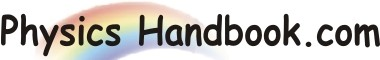HOME TOPICS DEFINITIONS TABLES LAWS INVENTIONS EXPERIMENTS QUIZ VIDEOS
 Wavelengths In Spectrum Of A Gas Aim : The aim of this experiment is to measure the wavelengths in the spectrum of a gas. Materials : -   A gas discharge lamp and transformer -   A spectrometer -   A dark room -   A diffraction grating -   Magnifying lens Procedure : -   Level the spectrometer table. -   Set up the spectrometer with the collimator adjusted to produce parallel light and the     telescope to receive it. -   Put the grating on the spectrometer table at right angles to the axis and facing towards the     telescope. -   Measure the angle between a given line in the first order on either side of the central     maximum. Half of this angle will give the angle of diffraction (A). -   Repeat this for as many lines as can be seen. -  Record the number of line per metre (N) on the diffraction grating and hence find the grating     spacing (e = 1/N) Conclusion : You can calculate the wavelengths (λ) from n λ = esinA. where, n is the order of the spectrum